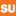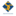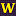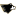Mathematics Commons™

17,824 Full-Text Articles 17,097 Authors 4,137,002 Downloads285 Institutions

All Articles in Mathematics

17,824 full-text articles. Page 456 of 526.

Pt-Symmetric Oligomers: Analytical Solutions, Linear Stability, And Nonlinear Dynamics, 2011UMass, Amherst

Pt-Symmetric Oligomers: Analytical Solutions, Linear Stability, And Nonlinear Dynamics, K. Li, Panos Kevrekidis

Panos Kevrekidis

In the present work we focus on the case of (few-site) configurations respecting the parity-time (PT) symmetry, i.e., with a spatially odd gain-loss profile. We examine the case of such “oligomers” with not only two sites, as in earlier works, but also the cases of three and four sites. While in the former case of recent experimental interest the picture of existing stationary solutions and their stability is fairly straightforward, the latter cases reveal a considerable additional complexity of solutions, including ones that exist past the linear PT-symmetry breaking point in the case of the trimer, and symmetry-breaking bifurcations ...

The Time At Which A Lévy Process Creeps, 2011Syracuse University

The Time At Which A Lévy Process Creeps, Philip S. Griffin, Ross A. Maller

Mathematics - Faculty Scholarship

We show that if a Levy process creeps then, as a function of u, the renewal function V (t, u) of the bivariate ascending ladder process (L−1,H) is absolutely continuous on [0,∞) and left differentiable on (0,∞), and the left derivative at u is proportional to the (improper) distribution function of the time at which the process creeps over level u, where the constant of proportionality is d−1H, the reciprocal of the (positive) drift of H. This yields the (missing) term due to creeping in the recent quintuple law of Doney and Kyprianou (2006). As an ...

Path Decomposition Of Ruinous Behaviour For A General Lévy Insurance Risk Process, 2011Syracuse University

Path Decomposition Of Ruinous Behaviour For A General Lévy Insurance Risk Process, Philip S. Griffin, Ross A. Maller

Mathematics - Faculty Scholarship

We analyse the general Levy insurance risk process for Levy measures in the convolution equivalence class

S(alpha), alpha> 0, via a new kind of path decomposition. This yields a very general functional limit theorem as the initial reserve level u → ∞, and a host of new results for functionals of interest in insurance risk. Particular emphasis is placed on the time to ruin, which is shown to have a proper limiting distribution, as u → ∞, conditional on ruin occurring, under our assumptions. Existing asymptotic results under the S(alpha)

assumption are synthesised and extended, and proofs are much simplified, by comparison ...

Path Decomposition Of Ruinous Behaviour For A General Lévy Insurance Risk Process, 2011Syracuse University

Path Decomposition Of Ruinous Behaviour For A General Lévy Insurance Risk Process, Philip S. Griffin, Ross A. Maller

Mathematics - Faculty Scholarship

We analyse the general Levy insurance risk process for Levy measures in the convolution equivalence class S(alpha), alpha > 0, via a new kind of path decomposition. This yields a very general functional limit theorem as the initial reserve level u → ∞, and a host of new results for functionals of interest in insurance risk. Particular emphasis is placed on the time to ruin, which is shown to have a proper limiting distribution, as u -> ∞, conditional on ruin occurring, under our assumptions. Existing asymptotic results under the S(alpha) assumption are synthesised and extended, and proofs are much simplified, by comparison ...

A Positive Monk Formula In The S1-Equivariant Cohomology Of Type A Peterson Varieties, 2011McMaster University

A Positive Monk Formula In The S1-Equivariant Cohomology Of Type A Peterson Varieties, Megumi Harada, Julianna Tymoczko

Mathematics and Statistics: Faculty Publications

Peterson varieties are a special class of Hessenberg varieties that have been extensively studied e.g. by Peterson, Kostant, and Rietsch, in connection with the quantum cohomology of the flag variety. In this manuscript, we develop a generalized Schubert calculus, and in particular a positive Chevalley-Monk formula, for the ordinary and Borel-equivariant cohomology of the Peterson variety Y in type An−1, with respect to a natural S1 -action arising from the standard action of the maximal torus on flag varieties. As far as we know, this is the first example of positive Schubert calculus beyond the realm ...

Nonlinear Excitations, Stability Inversions, And Dissipative Dynamics In Quasi-One-Dimensional Polariton Condensates, 2011UMass, Amherst

Nonlinear Excitations, Stability Inversions, And Dissipative Dynamics In Quasi-One-Dimensional Polariton Condensates, J. Cuevas, A. S. Rodrigues, R. Carretero-Gonz´Alez, Panos Kevrekidis, D. J. Frantzeskakis

Panos Kevrekidis

We study the existence, stability, and dynamics of the ground state and nonlinear excitations, in the form of dark solitons, for a quasi-one-dimensional polariton condensate in the presence of nonresonant pumping and nonlinear damping. We find a series of remarkable features that can be directly contrasted to the case of the typically energy-conserving ultracold alkali-atom Bose-Einstein condensates. For some sizable parameter ranges, the nodeless (“ground”) state becomes unstabletoward the formation of stable nonlinear single- or multi-dark-soliton excitations. It is also observed that for suitable parametric choices, the instability of single dark solitons can nucleate multi-dark-soliton states. Also, for other parametric ...

The Time At Which A Lévy Process Creeps, 2011Syracuse University

The Time At Which A Lévy Process Creeps, Philip S. Griffin, Ross A. Maller

Mathematics - Faculty Scholarship

We show that if a Levy process creeps then, as a function of u, the renewal function V (t, u) of the bivariate ascending ladder process (L−1,H) is absolutely continuous on [0,∞) and left differentiable on (0,∞), and the left derivative at u is proportional to the (improper) distribution function of the time at which the process creeps over level u, where the constant of proportionality is d−1H , the reciprocal of the (positive) drift of H. This yields the (missing) term due to creeping in the recent quintuple law of Doney and Kyprianou (2006). As an ...

Polynomial Generalizations Of Two-Variable Ramanujan Type Identities, 2011West Chester University of Pennsylvania

Polynomial Generalizations Of Two-Variable Ramanujan Type Identities, James Mclaughlin, Andrew V. Sills

Mathematics

No abstract provided.

Hamilton-Waterloo Problem With Triangle And C9 Factors, 2011Michigan Technological University

Hamilton-Waterloo Problem With Triangle And C9 Factors, David C. Kamin

Dissertations, Master's Theses and Master's Reports - Open

The Hamilton-Waterloo problem and its spouse-avoiding variant for uniform cycle sizes asks if Kv, where v is odd (or Kv - F, if v is even), can be decomposed into 2-factors in which each factor is made either entirely of m-cycles or entirely of n-cycles. This thesis examines the case in which r of the factors are made up of cycles of length 3 and s of the factors are made up of cycles of length 9, for any r and s. We also discuss a constructive solution to the general (m,n) case which fixes r and s.

The Onset Of Oscillations In Microvascular Blood Flow, 2011Franklin W. Olin College of Engineering

The Onset Of Oscillations In Microvascular Blood Flow, John B. Geddes, Russell T. Carr, Nathaniel J. Karst, Fan Wu

John B. Geddes

We explore the stability of equilibrium solution(s) of a simple model of microvascular blood flow in a two-node network. The model takes the form of convection equations for red blood cell concentration, and contains two important rheological effects—the Fåhræus–Lindqvist effect, which governs viscosity of blood flow in a single vessel, and the plasma skimming effect, which describes the separation of red blood cells at diverging nodes. We show that stability is governed by a linear system of integral equations, and we study the roots of the associated characteristic equation in detail. We demonstrate using a combination of ...

Pulse Dynamics In An Actively Mode-Locked Laser, 2011Franklin W. Olin College of Engineering

Pulse Dynamics In An Actively Mode-Locked Laser, John Geddes, Willie Firth, Kelly Black

John B. Geddes

We consider pulse formation dynamics in an actively mode-locked laser. We show that an amplitude-modulated laser is subject to large transient growth and we demonstrate that at threshold the transient growth is precisely the Petermann excess noise factor for a laser governed by a nonnormal operator. We also demonstrate an exact reduction from the governing PDEs to a low-dimensional system of ODEs for the parameters of an evolving pulse. A linearized version of these equations allows us to find analytical expressions for the transient growth below threshold. We also show that the nonlinear system collapses onto an appropriate fixed point ...

Distinguishability Of Infinite Groups And Graphs, 2011Syracuse University

Distinguishability Of Infinite Groups And Graphs, Simon M. Smith, Thomas W. Tucker, Mark E. Watkins

Mathematics - Faculty Scholarship

The distinguishing number of a group G acting faithfully on a set V is the least number of colors needed to color the elements of V so that no non-identity element of the group preserves the coloring. The distinguishing number of a graph is the distinguishing number of its full automorphism group acting on its vertex set. A connected graph T is said to have connectivity 1 if there exists a vertex alpha in VT such that T \ {alpha} is not connected. For alpha in V , an orbit of the point stabilizer Galpha is called a suborbit of G ...

Estimation Algorithm For Mixture Of Experts Recurrent Event Model, 2011Georgia State University

Estimation Algorithm For Mixture Of Experts Recurrent Event Model, Timesha U. Brooks

Mathematics Theses

﻿﻿﻿﻿This paper proposes a mixture of experts recurrent events model. This general model accommodates an unobservable frailty variable, intervention effect, influence of accumulating event occurrences, and covariate effects. A latent class variable is utilized to deal with a heterogeneous population and associated covariates. A homogeneous nonparametric baseline hazard and heterogeneous parametric covariate effects are assumed. Maximum likelihood principle is employed to obtain parameter estimates. Since the frailty variable and latent classes are unobserved, an estimation procedure is derived through the EM algorithm. A simulated data set is generated to illustrate the data structure of recurrent events for a heterogeneous population.

2011Boise State University

Inducing Normality From Non-Gaussian Long Memory Time Series And Its Application To Stock Return Data, Kyungduk Ko

Kyungduk Ko

Motivated by Lee and Ko (Appl. Stochastic Models. Bus. Ind. 2007; 23:493–502) but not limited to the study, this paper proposes a wavelet-based Bayesian power transformation procedure through the well-known Box–Cox transformation to induce normality from non-Gaussian long memory processes. We consider power transformations of non-Gaussian long memory time series under the assumption of an unknown transformation parameter, a situation that arises commonly in practice, while most research has been devoted to non-linear transformations of Gaussian long memory time series with known transformation parameter. Specially, this study is mainly focused on the simultaneous estimation of the transformation ...

A Multi-Frequency Inverse Source Problem For The Helmholtz Equation, 2011Brigham Young University - Provo

A Multi-Frequency Inverse Source Problem For The Helmholtz Equation, Sebastian Ignacio Acosta

Theses and Dissertations

The inverse source problem for the Helmholtz equation is studied. An unknown source is to be identified from the knowledge of its radiated wave. The focus is placed on the effect that multi-frequency data has on establishing uniqueness. In particular, we prove that data obtained from finitely many frequencies is not sufficient. On the other hand, if the frequency varies within an open interval of the positive real line, then the source is determined uniquely. An algorithm is based on an incomplete Fourier transform of the measured data and we establish an error estimate under certain regularity assumptions on the ...

2011Brigham Young University - Provo

Three-Dimensional Galois Representations And A Conjecture Of Ash, Doud, And Pollack, Vinh Xuan Dang

Theses and Dissertations

In the 1970s and 1980s, Jean-Pierre Serre formulated a conjecture connecting two-dimensional Galois representations and modular forms. The conjecture came to be known as Serre's modularity conjecture. It was recently proved by Khare and Wintenberger in 2008. Serre's conjecture has various important consequences in number theory. Most notably, it played a key role in the proof of Fermat's last theorem. A natural question is, what is the analogue of Serre's conjecture for higher dimensional Galois representations? In 2002, Ash, Doud and Pollack formulated a precise statement for a higher dimensional analogue of Serre's conjecture. They ...

2011China University of Mining & Technology

Applications Of Local Fractional Calculus To Engineering In Fractal Time-Space: Local Fractional Differential Equations With Local Fractional Derivative, Yang Xiao-Jun

Xiao-Jun Yang

This paper presents a better approach to model an engineering problem in fractal-time space based on local fractional calculus. Some examples are given to elucidate to establish governing equations with local fractional derivative.

2011China University of Mining & Technology

A Short Introduction To Local Fractional Complex Analysis, Yang Xiao-Jun

Xiao-Jun Yang

This paper presents a short introduction to local fractional complex analysis. The generalized local fractional complex integral formulas, Yang-Taylor series and local fractional Laurent’s series of complex functions in complex fractal space, and generalized residue theorems are investigated.

Dark-Bright Gap Solitons In Coupled-Mode One-Dimensional Saturable Waveguide Arrays, 2011UMASS, Amherst

Dark-Bright Gap Solitons In Coupled-Mode One-Dimensional Saturable Waveguide Arrays, Rong Dong, Christian E. Ruter, Detlef Kip, Jesus Cuevas, Panos Kevrekidis, Daohong Song, Jingjun Xu

Panos Kevrekidis

In the present work, we consider the dynamics of dark solitons as one mode of a defocusing photorefractive lattice coupled with bright solitons as a second mode of the lattice. Our investigation is motivated by an experiment that illustrates that such coupled states can exist with both components in the first gap of the linear band spectrum. This finding is further extended by the examination of different possibilities from a theoretical perspective, such as symbiotic ones where the bright component is supported by states of the dark component in the first or second gap, or nonsymbiotic ones where the bright ...

2011China University of Mining & Technology

Fractional Trigonometric Functions In Complex-Valued Space: Applications Of Complex Number To Local Fractional Calculus Of Complex Function, Yang Xiao-Jun

Xiao-Jun Yang

This paper presents the fractional trigonometric functions in complex-valued space and proposes a short outline of local fractional calculus of complex function in fractal spaces.